Anzeige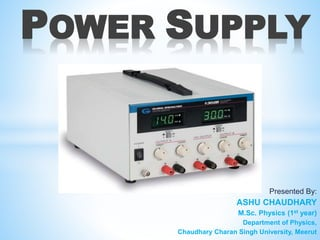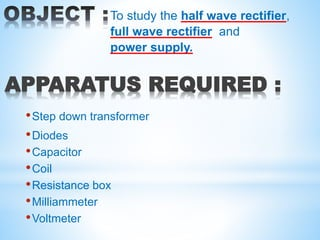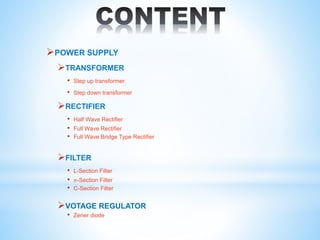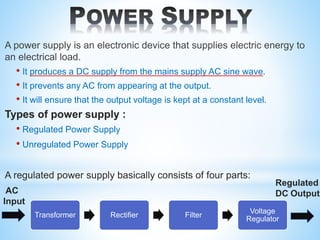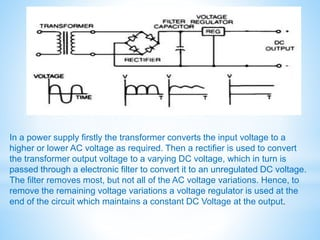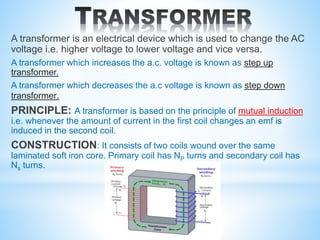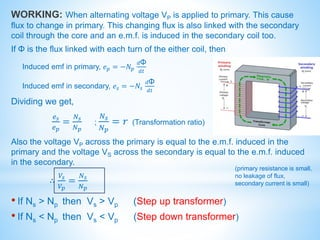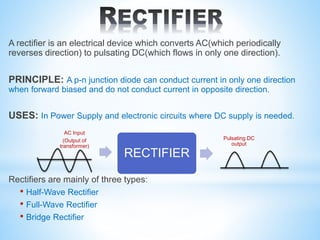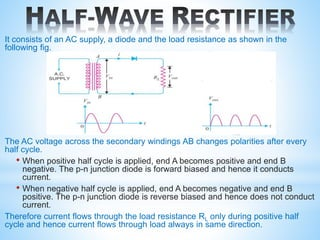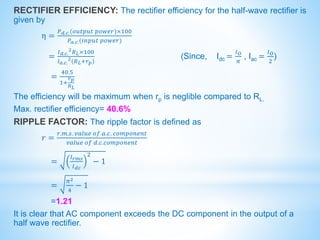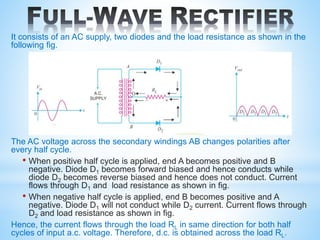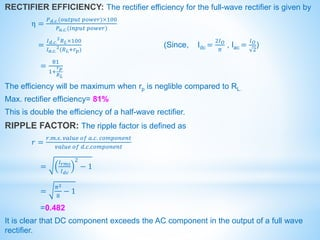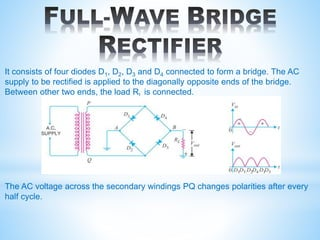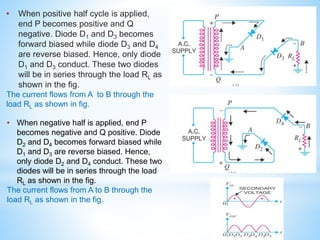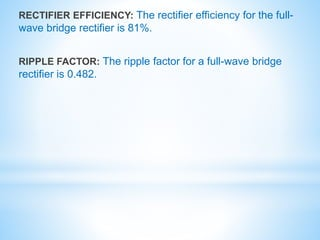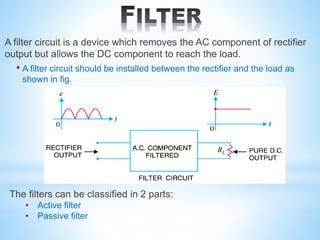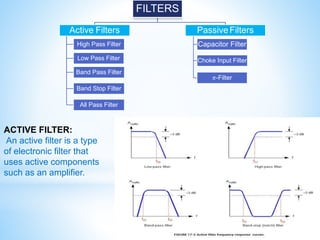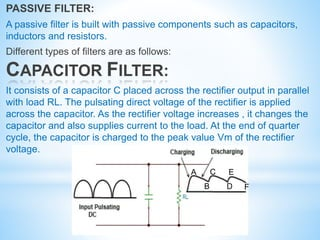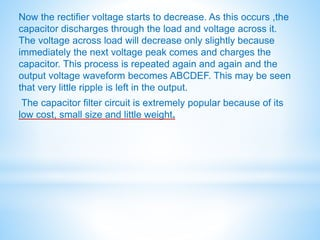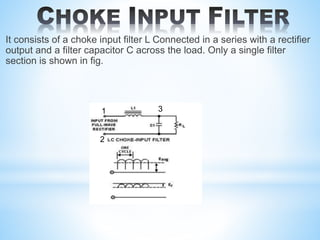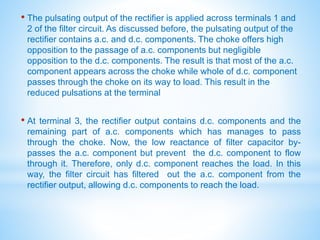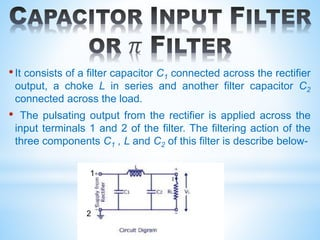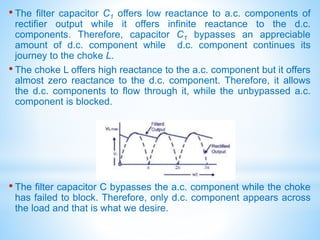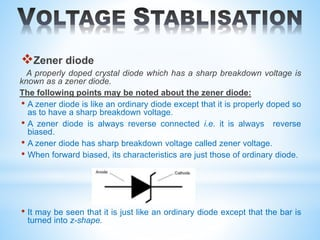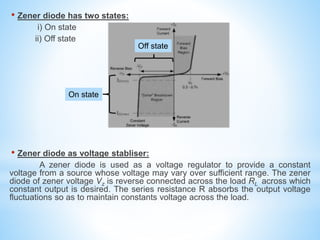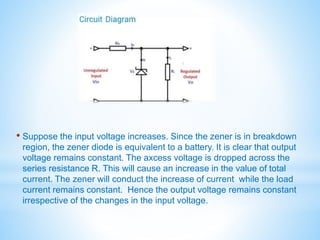1 von 27
Anzeige

### POWER SUPPLY(Ashu).pptx

1. Presented By: ASHU CHAUDHARY M.Sc. Physics (1st year) Department of Physics, Chaudhary Charan Singh University, Meerut POWER SUPPLY
2. APPARATUS REQUIRED : •Step down transformer •Diodes •Capacitor •Coil •Resistance box •Milliammeter •Voltmeter To study the half wave rectifier, full wave rectifier and power supply.
3. POWER SUPPLY TRANSFORMER • Step up transformer • Step down transformer RECTIFIER • Half Wave Rectifier • Full Wave Rectifier • Full Wave Bridge Type Rectifier FILTER • L-Section Filter • 𝜋-Section Filter • C-Section Filter VOTAGE REGULATOR • Zener diode
4. A power supply is an electronic device that supplies electric energy to an electrical load. • It produces a DC supply from the mains supply AC sine wave. • It prevents any AC from appearing at the output. • It will ensure that the output voltage is kept at a constant level. Types of power supply : • Regulated Power Supply • Unregulated Power Supply A regulated power supply basically consists of four parts: Transformer Rectifier Filter Voltage Regulator Regulated DC Output AC Input
5. In a power supply firstly the transformer converts the input voltage to a higher or lower AC voltage as required. Then a rectifier is used to convert the transformer output voltage to a varying DC voltage, which in turn is passed through a electronic filter to convert it to an unregulated DC voltage. The filter removes most, but not all of the AC voltage variations. Hence, to remove the remaining voltage variations a voltage regulator is used at the end of the circuit which maintains a constant DC Voltage at the output.
6. A transformer is an electrical device which is used to change the AC voltage i.e. higher voltage to lower voltage and vice versa. A transformer which increases the a.c. voltage is known as step up transformer. A transformer which decreases the a.c voltage is known as step down transformer. PRINCIPLE: A transformer is based on the principle of mutual induction i.e. whenever the amount of current in the first coil changes an emf is induced in the second coil. CONSTRUCTION: It consists of two coils wound over the same laminated soft iron core. Primary coil has NP turns and secondary coil has Ns turns.
7. WORKING: When alternating voltage VP is applied to primary. This cause flux to change in primary. This changing flux is also linked with the secondary coil through the core and an e.m.f. is induced in the secondary coil too. If Φ is the flux linked with each turn of the either coil, then Induced emf in primary, 𝑒𝑝 = −𝑁𝑝 𝑑Φ 𝑑𝑡 Induced emf in secondary, 𝑒𝑠 = −𝑁𝑠 𝑑Φ 𝑑𝑡 Dividing we get, 𝑒𝑠 𝑒𝑝 = 𝑁𝑠 𝑁𝑝 ; 𝑁𝑠 𝑁𝑝 = 𝑟 (Transformation ratio) Also the voltage VP across the primary is equal to the e.m.f. induced in the primary and the voltage VS across the secondary is equal to the e.m.f. induced in the secondary. ∴ 𝑉𝑠 𝑉𝑝 = 𝑁𝑠 𝑁𝑝 • If Ns ˃ Np then Vs ˃ Vp (Step up transformer) • If Ns ˂ Np then Vs ˂ Vp (Step down transformer) (primary resistance is small, no leakage of flux, secondary current is small)
8. A rectifier is an electrical device which converts AC(which periodically reverses direction) to pulsating DC(which flows in only one direction). PRINCIPLE: A p-n junction diode can conduct current in only one direction when forward biased and do not conduct current in opposite direction. USES: In Power Supply and electronic circuits where DC supply is needed. Rectifiers are mainly of three types: • Half-Wave Rectifier • Full-Wave Rectifier • Bridge Rectifier AC Input (Output of transformer) RECTIFIER Pulsating DC output
9. It consists of an AC supply, a diode and the load resistance as shown in the following fig. The AC voltage across the secondary windings AB changes polarities after every half cycle. • When positive half cycle is applied, end A becomes positive and end B negative. The p-n junction diode is forward biased and hence it conducts current. • When negative half cycle is applied, end A becomes negative and end B positive. The p-n junction diode is reverse biased and hence does not conduct current. Therefore current flows through the load resistance RL only during positive half cycle and hence current flows through load always in same direction. A B
10. RECTIFIER EFFICIENCY: The rectifier efficiency for the half-wave rectifier is given by η = 𝑃𝑑.𝑐.(𝑜𝑢𝑡𝑝𝑢𝑡 𝑝𝑜𝑤𝑒𝑟)×100 𝑃𝑎.𝑐.(𝑖𝑛𝑝𝑢𝑡 𝑝𝑜𝑤𝑒𝑟) = 𝐼𝑑.𝑐. 2𝑅𝐿×100 𝐼𝑎.𝑐. 2(𝑅𝐿+𝑟𝑝) (Since, Idc = 𝐼𝑂 𝜋 , Iac = 𝐼𝑂 2 ) = 40.5 1+ 𝑟𝑝 𝑅𝐿 The efficiency will be maximum when rp is neglible compared to RL. Max. rectifier efficiency= 40.6% RIPPLE FACTOR: The ripple factor is defined as 𝑟 = 𝑟.𝑚.𝑠. 𝑣𝑎𝑙𝑢𝑒 𝑜𝑓 𝑎.𝑐. 𝑐𝑜𝑚𝑝𝑜𝑛𝑒𝑛𝑡 𝑣𝑎𝑙𝑢𝑒 𝑜𝑓 𝑑.𝑐.𝑐𝑜𝑚𝑝𝑜𝑛𝑒𝑛𝑡 = 𝐼𝑟𝑚𝑠 𝐼𝑑𝑐 2 − 1 = 𝜋2 4 − 1 =1.21 It is clear that AC component exceeds the DC component in the output of a half wave rectifier.
11. It consists of an AC supply, two diodes and the load resistance as shown in the following fig. The AC voltage across the secondary windings AB changes polarities after every half cycle. • When positive half cycle is applied, end A becomes positive and B negative. Diode D1 becomes forward biased and hence conducts while diode D2 becomes reverse biased and hence does not conduct. Current flows through D1 and load resistance as shown in fig. • When negative half cycle is applied, end B becomes positive and A negative. Diode D1 will not conduct while D2 current. Current flows through D2 and load resistance as shown in fig. Hence, the current flows through the load RL in same direction for both half cycles of input a.c. voltage. Therefore, d.c. is obtained across the load RL.
12. RECTIFIER EFFICIENCY: The rectifier efficiency for the full-wave rectifier is given by η = 𝑃𝑑.𝑐.(𝑜𝑢𝑡𝑝𝑢𝑡 𝑝𝑜𝑤𝑒𝑟)×100 𝑃𝑎.𝑐.(𝑖𝑛𝑝𝑢𝑡 𝑝𝑜𝑤𝑒𝑟) = 𝐼𝑑.𝑐. 2 𝑅𝐿×100 𝐼𝑎.𝑐. 2 (𝑅𝐿+𝑟𝑝) (Since, Idc = 2𝐼𝑂 𝜋 , Iac = 𝐼𝑂 2 ) = 81 1+ 𝑟𝑝 𝑅𝐿 The efficiency will be maximum when rp is neglible compared to RL. Max. rectifier efficiency= 81% This is double the efficiency of a half-wave rectifier. RIPPLE FACTOR: The ripple factor is defined as 𝑟 = 𝑟.𝑚.𝑠. 𝑣𝑎𝑙𝑢𝑒 𝑜𝑓 𝑎.𝑐. 𝑐𝑜𝑚𝑝𝑜𝑛𝑒𝑛𝑡 𝑣𝑎𝑙𝑢𝑒 𝑜𝑓 𝑑.𝑐.𝑐𝑜𝑚𝑝𝑜𝑛𝑒𝑛𝑡 = 𝐼𝑟𝑚𝑠 𝐼𝑑𝑐 2 − 1 = 𝜋2 8 − 1 =0.482 It is clear that DC component exceeds the AC component in the output of a full wave rectifier.
13. It consists of four diodes D1, D2, D3 and D4 connected to form a bridge. The AC supply to be rectified is applied to the diagonally opposite ends of the bridge. Between other two ends, the load RL is connected. The AC voltage across the secondary windings PQ changes polarities after every half cycle.
14. • When positive half cycle is applied, end P becomes positive and Q negative. Diode D1 and D3 becomes forward biased while diode D3 and D4 are reverse biased. Hence, only diode D1 and D3 conduct. These two diodes will be in series through the load RL as shown in the fig. The current flows from A to B through the load RL as shown in fig. • When negative half is applied, end P becomes negative and Q positive. Diode D2 and D4 becomes forward biased while D1 and D3 are reverse biased. Hence, only diode D2 and D4 conduct. These two diodes will be in series through the load RL as shown in the fig. The current flows from A to B through the load RL as shown in the fig.
15. RECTIFIER EFFICIENCY: The rectifier efficiency for the full- wave bridge rectifier is 81%. RIPPLE FACTOR: The ripple factor for a full-wave bridge rectifier is 0.482.
16. A filter circuit is a device which removes the AC component of rectifier output but allows the DC component to reach the load. • A filter circuit should be installed between the rectifier and the load as shown in fig. The filters can be classified in 2 parts: • Active filter • Passive filter
17. FILTERS Active Filters High Pass Filter Low Pass Filter Band Pass Filter Band Stop Filter All Pass Filter PassiveFilters Capacitor Filter Choke Input Filter 𝜋-Filter ACTIVE FILTER: An active filter is a type of electronic filter that uses active components such as an amplifier.
18. PASSIVE FILTER: A passive filter is built with passive components such as capacitors, inductors and resistors. Different types of filters are as follows: CAPACITOR FILTER: It consists of a capacitor C placed across the rectifier output in parallel with load RL. The pulsating direct voltage of the rectifier is applied across the capacitor. As the rectifier voltage increases , it changes the capacitor and also supplies current to the load. At the end of quarter cycle, the capacitor is charged to the peak value Vm of the rectifier voltage. A B C D E F
19. Now the rectifier voltage starts to decrease. As this occurs ,the capacitor discharges through the load and voltage across it. The voltage across load will decrease only slightly because immediately the next voltage peak comes and charges the capacitor. This process is repeated again and again and the output voltage waveform becomes ABCDEF. This may be seen that very little ripple is left in the output. The capacitor filter circuit is extremely popular because of its low cost, small size and little weight.
20. It consists of a choke input filter L Connected in a series with a rectifier output and a filter capacitor C across the load. Only a single filter section is shown in fig. 1 2 3
21. • The pulsating output of the rectifier is applied across terminals 1 and 2 of the filter circuit. As discussed before, the pulsating output of the rectifier contains a.c. and d.c. components. The choke offers high opposition to the passage of a.c. components but negligible opposition to the d.c. components. The result is that most of the a.c. component appears across the choke while whole of d.c. component passes through the choke on its way to load. This result in the reduced pulsations at the terminal • At terminal 3, the rectifier output contains d.c. components and the remaining part of a.c. components which has manages to pass through the choke. Now, the low reactance of filter capacitor by- passes the a.c. component but prevent the d.c. component to flow through it. Therefore, only d.c. component reaches the load. In this way, the filter circuit has filtered out the a.c. component from the rectifier output, allowing d.c. components to reach the load.
22. •It consists of a filter capacitor C1 connected across the rectifier output, a choke L in series and another filter capacitor C2 connected across the load. • The pulsating output from the rectifier is applied across the input terminals 1 and 2 of the filter. The filtering action of the three components C1 , L and C2 of this filter is describe below- 1 2
23. • The filter capacitor C1 offers low reactance to a.c. components of rectifier output while it offers infinite reactance to the d.c. components. Therefore, capacitor C1 bypasses an appreciable amount of d.c. component while d.c. component continues its journey to the choke L. • The choke L offers high reactance to the a.c. component but it offers almost zero reactance to the d.c. component. Therefore, it allows the d.c. components to flow through it, while the unbypassed a.c. component is blocked. • The filter capacitor C bypasses the a.c. component while the choke has failed to block. Therefore, only d.c. component appears across the load and that is what we desire.
24. Zener diode A properly doped crystal diode which has a sharp breakdown voltage is known as a zener diode. The following points may be noted about the zener diode: • A zener diode is like an ordinary diode except that it is properly doped so as to have a sharp breakdown voltage. • A zener diode is always reverse connected i.e. it is always reverse biased. • A zener diode has sharp breakdown voltage called zener voltage. • When forward biased, its characteristics are just those of ordinary diode. • It may be seen that it is just like an ordinary diode except that the bar is turned into z-shape.
25. • Zener diode has two states: i) On state ii) Off state • Zener diode as voltage stabliser: A zener diode is used as a voltage regulator to provide a constant voltage from a source whose voltage may vary over sufficient range. The zener diode of zener voltage Vz is reverse connected across the load RL across which constant output is desired. The series resistance R absorbs the output voltage fluctuations so as to maintain constants voltage across the load. On state Off state
26. • Suppose the input voltage increases. Since the zener is in breakdown region, the zener diode is equivalent to a battery. It is clear that output voltage remains constant. The axcess voltage is dropped across the series resistance R. This will cause an increase in the value of total current. The zener will conduct the increase of current while the load current remains constant. Hence the output voltage remains constant irrespective of the changes in the input voltage.
Anzeige